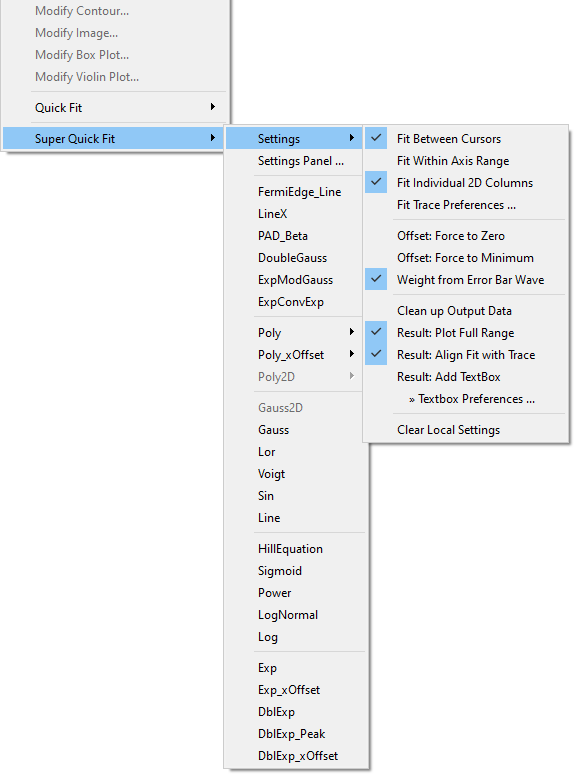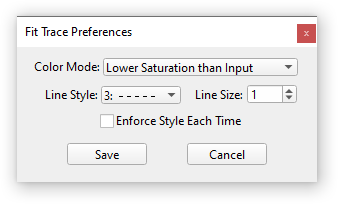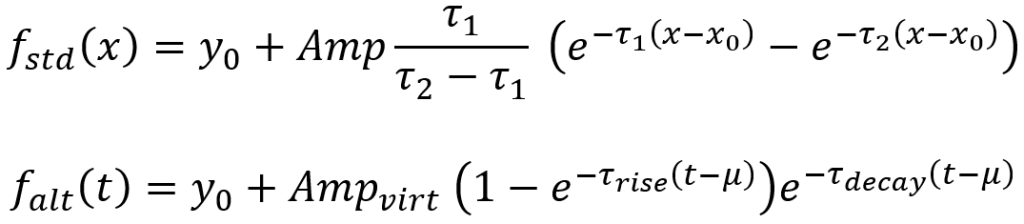# Super Quick Fit - Quick Fit with more options, derived values output and user-function support

This project supplants the built-in Quick Fit, which is user to quickly fit data displayed in a graph. Super Quick Fit provides all functionality of Quick Fit and adds several convenient features.

Special thanks to Oleg Dyachok from Uppsala University for suggesting and testing improvements for version 1.05 and beyond.

I tried my best to test everything thoroughly, but sometimes bugs slip through. Bug reports and suggestions for new features are always welcome. I am happy to add more useful settings, peak shapes or derived values if I receive feedback. Currently, 2D fit support may not be perfect since I don't have any meaningful data to test everything. If you have suggestions for improvement of 2D fitting or can test this functionality in detail, then please let me know.### More settings:

• Fit Between Cursors - Same as for Quick Fit. The fit range is constrained by the cursors.
• Fit Within Axis Range - The fit range is constrained to the current top / bottom (and for images left / right) axis scale of the graph.
• Fit Individual 2D Columns - If the graph contains traces from a 2D wave, then each fitted column gets its own output wave named with the prefix 'fit_XX_'. XX designates the fitted column where the number of digits depends on the y-size of the wave (e.g., a wave with 200 columns would give 'fit_000_wavename' as output for the first column).
• Fit Trace Preferences - Opens a panel to choose the preferred fit-trace style (see below)
• Offset: Force to Zero - The 'offset value' of the fit function (most often the baseline value y0) is held at zero.
• Offset: Force to Minimum - The 'offset value' held at a minimum. The minimum is either determined by the minimum value from placed cursors or the minimum of the data (depending on the fit function this could be either a x or y minimum).
• Weight from Error Bar Wave - Same as for Quick Fit. The weight wave is taken from the error-bar wave of the trace.
• Clean up Output Data - Deletes output waves (other than the 'fit_' wave), strings and variables after the fit. Use this if you are only interested in values printed in the history (and the graph's text box) and otherwise want to keep folders from accumulating W_coef, W_sigma waves etc..
• Result: Plot Full Range - The fit is plotted over the full axis range of the graph.
• Result: Align Fit with Trace - The fit trace is scaled and offset in the same way as the source trace after the fit to align the fit trace to the source.
• Result: Add TextBox - Adds a standard text box with results to the graph just like the standard Quick Fit.
• Textbox Preferences - Same as for Quick Fit. Choose what you would like to have printed into the result textbox.

Settings are saved in the current experiment (as the string root:QuickFitMenu_settings) to keep used settings consistent for each experiment file. But the last-used settings are also persistent and are loaded for each new Igor session, which makes it possible to set up your favorite settings and keep using them. Choose Clear Local Settings to reset any persistent local setting (i.e., QuickFitMenu_settings) and load the global settings instead.Fit Trace Preferences lets you set your preferred colors and line style for newly added fit traces after the fit. This helps to make traces more distinctive against the source trace. Note that already plotted fit traces are not changed even if the fit is redone to preserve the style of traces.

(All functions other than EMG are also usable in the Curve-Fit dialog)

• FermiEdge_Line - Fermi–Dirac distribution combined with a linear slope.
Equation: f(E) = y0 + (m*E+A) / (exp((E-Ef)/kT)+1)

• LineX - A line defined with the x-intercept (x0) as direct coefficient. Use this function if you want to determine x-intercepts with a low uncertainty, especially of lines with steep slope. The normal Line function is instead based on y-intercepts and bad at finding x-intercepts.
Equation: f(x) = m*(x-x0)

• PAD_Beta - Angular distribution function, e.g., for analyzing photoemission experiments.
Equation: f(theta) = Scale*(1+Beta*legendreA(2,0,cos((theta-theta_0)*(Pi/180))))

• DoubleGauss - A combination of two Gaussian peaks to fit doublets etc. This fit will likely not work well if your data range does not contain two clear 'peak' features.
Equation: f(x) = y0 + Area1 * Gauss(x,Loc1,Width1) + Area2 * Gauss(x,Loc2,Width2)

• ExpModGauss - Exponentially Modified Gaussian (EMG) peak shape. A often-used asymmetric peak shape. Equal to the same function offered in the Multipeak Fit package.

• ExpConvExp - Convolution of two exponential decay functions. Equal to the same function offered in the Multipeak Fit package.

### Output of Derived Values:

Additional parameters such as FWHM, area, height etc. (where applicable) are printed in history and the graph's text box. Currently, the following functions output derived parameters: LineX, DoubleGauss, ExpModGauss, ExpConvExp, Line, Gauss, Lor(entz), and Voigt. You can also set up your own function to output additional derived values after a fit (see below).

#### Alternative Derived Parameters for ExpConvExp:

The ExpConvExp function uses the standard functional form of the Multipeak Fit package, but derived parameters (virtual amplitude, inverse of the rise time, inverse of the decay time) for an alternative functional form are given at the same time. The alternative form is more common in some fields such as cell biology. Both functional forms are compared below:### Calling Super Quick Fit from the Command Line or Your Own Code:

Super Quick Fit can also be run in code by executing:

`SuperQuickFit(String graphName, String traceName, String fitFuncName)`

The graphName can be the name of any open graph or just "" for the top graph. traceName can also be left empty ("") to fit an image plot to the first trace in the trace list of the chosen graph. fitFuncName must be a valid built-in or user function name. SuperQuickFit() also returns error codes or 0 if the fit was successful. The fit settings are taken from the last saved settings and cannot overwritten at present. Set up everything to your liking in the right-click menu before running the function. This function is most useful as an easy way to fit multiple traces in a graph or traces in multiple graphs.

The error codes are:

``````-1 = no valid target or function
-2 = no graph found
-3 = designated graph does not exist
-4 = trace not on graph
-5 = not a valid function type
-10 = guess for fit function failed
-100 = image has separate x or y axis (not supported yet)
-999 = execution of the fit failed``````

Adding functions is easy. You only need a normal (such as created with the curve fit dialog) or all-at-once fit function and a second function which calculates the initial guesses. Let's look at a simple example, such as the LineX function included in the package:

Function LineX(Wave w, Variable x) : FitFunc
//CurveFitDialog/ Equation:
//CurveFitDialog/ f(x) = m*(x-x0)
//CurveFitDialog/ End of Equation
//CurveFitDialog/ Independent Variables 1
//CurveFitDialog/ x
//CurveFitDialog/ Coefficients 2
//CurveFitDialog/ w = x0
//CurveFitDialog/ w = m
return w*(x-w)
End

To create initial guesses and at the same time make this function appear in the Super Quick Fit menu, you need to provide a second function which has the name YourFitFuncName+"_prepareCoef", which has a special structure SuperQuickFitStruct as input. In our example the function needs to be called LineX_prepareCoef, like this:

Function LineX_prepareCoef(STRUCT SuperQuickFitStruct &s)
Make/D/O/N=2 W_coef = {0,1}
Wave s.cw = W_coef
return 0
End

The function needs to create a wave with the correct number of coefficients (2 in the example) and initial guesses which are likely make the fit succeed. The created coefficient wave then needs to be fed into the wave assignment s.cw. By the way, you don't have to call this wave W_coef. But if you choose this standard name, the coefficient wave will be properly deleted if you have the 'clean-up' setting active. Now you can select the function from the Super Quick Fit menu.

So far, the initial guesses are simply x0 = 0 and m = 1. This may be too simple for a good guess. You could, for example, access the input data to calculate more reasonable guesses. The full function for LineX looks like this:

Function LineX_prepareCoef(STRUCT SuperQuickFitStruct &s)
Duplicate/free/RMD=[][s.pntCol] s.data, dif     // support for 2D trace data via s.pntCol
Differentiate dif/D=dif
WaveStats/Q/R=[s.pntMin,s.pntMax] dif
Variable slope = (WaveMax(s.data) - WaveMin(s.data)) > 0 ? V_max : V_min
Make/D/O/N=2 W_coef = {(s.holdMode == 1 ? 0 : s.xBase),slope}
Wave s.cw = W_coef
return 0
End

Here, the slope m is taken from the derivative of the input data (=> wave s.data). Also, the offset x0 is calculated from the data provided by the fit kernel. Here, s.holdMode is the current offset setting (1 means offsets are forced to zero) and s.xBase is the current x minimum (either from the cursor or the minimum of the input data). This makes sure that the x0 coefficient is properly set to fix the line to the cursor or to zero.

By the way, the 'force offset' setting is by default applied to the first coefficient. If you want to hold other coefficients, you need to provide an appropriate hold string to s.holdStr (e.g., s.holdStr = "00001" fixes the fifth coefficient). The full structure definition looks like this:

Structure SuperQuickFitStruct
Wave cw     // coef wave
Wave sw     // covariance matrix (M_Covar)
Wave data   // data wave (1D or 2D)
Wave xw     // x wave
Wave yw     // y wave (for images; not supported yet)
int32 pntMin    // start point of fit range
int32 pntMax    // end point of fit range
int32 pntMinY   // start point in y direction (for images)
int32 pntMaxY   // end point  in y direction (for images)
double xBase    // x baseline / start offset
double yBase    // y baseline / start offset
int16 holdMode  // current hold setting:  = no hold,  = force o zero,  = force to min
int16 plotFull  // setting for plot over full range
int16 doTextbox // setting for text-box drawing
String holdStr  // hold string for the fit
EndStructure

Super Quick Fit supports additional data processing after the fit is done, such as calculating derived values from the fit results. For this, create a function named YourFitFuncName+"_derivedVals". In principle, you can do anything in this function, but the main purpose was intended to return a string to print into the history. Let's again look at an example (from LineX):

Function/S LineX_derivedVals(STRUCT SuperQuickFitStruct &s)
Variable yCross = -s.cw*s.cw
Variable yCross_err = yCross * sqrt( (s.sw/s.cw^2) + (s.sw/s.cw^2) + 2*s.sw/(s.cw*s.cw) )
String printStr = ""
sPrintf printStr, "Derived values:\r\tY-intercept\t= %g ± %g\r", yCross, (numtype(yCross_err) != 0 ? 0 : yCross_err)
if (s.doTextbox)
AppendText "\t"+printStr
endif
return printStr
End

This function calculates the y-intercept for the line from the fit coefficients (s.cw) and the covariance matrix (s.sw) and then prepares an output string printStr with the result. Here, the result is also added to the default text box, but you can of course omit this step. All available data is again received from the same SuperQuickFitStruct. You can also add code for a follow-up analysis of the result, saving of the data to disk or whatever you like here. Basically, you can add anything you want to have done after invoking the fit from the menu.

#### Generic Derived-Values Function:

If instead, you would rather do post-processing every time Super Quick Fit runs successfully, you can also write a generic (fit-function agnostic) function. This function must have the fixed name "SuperQuickFit_derivedVals", but does otherwise work exactly the same as the fit-function specific version explained above. Note that if both a generic and a fit-function specific post-processing function is present, then the generic function runs last. Below function demonstrates how this works. The function simply adds a short sentence to the results annotation. Again, there are no restrictions other that the function should not use Abort to halt the successful completion of Super Quick Fit itself.

function/S SuperQuickFit_derivedVals(STRUCT SuperQuickFitStruct &s)
if (s.doTextbox)
AppendText "This is a test."
endif
return ""
end

## Current Project Release

 Release File: Version: IGOR.8.00.x-1.12 Version Date: Fri, 11/24/2023 - 01:45 am Version Major: 1 Version Patch Level: 12 OS Compatibility: Windows Mac-Intel Release Notes: Fixed bug: Local settings could not be cleared. Fixed bug: custom fit functions did not fully support 2D x-waves. Added panel for global settings. Introduced pntCol_xw to designate column of a 2D x-wave for fitting. Now generic (fit-func agnostic) post-processing is executed, when a function with the name SuperQuickFit_derivedVals() exists. More robust handling of derivedVals functions. Added option to enforce fit trace style. Fitting individual columns now creates separate result annotations for each column. Correct trace markers are displayed now inside annotations when 'Fit Individual 2D Columns' is active.

John, thank you for your comment. I am happy that you found this interesting. At first, I just wanted to add in some functions to the Quick Fit menu, but it was not possible to modify the official menu structure. The I started my own submenu, which quickly grew into this full 'replacement'. :) Would be great if some features would find their way into the official Quick Fit at some point. I think not many people will find this package after all.

Looking at the screen shots, and reading some of the description, it is clear you have added user-define fit functions. You must also have some auto-guessing code for those functions. The reason the built-in Quick Fit menu doesn't list any user-defined functions is the lack of a way to provide auto-guessing. So I guess that would be a necessary enhancement to make that a possibility.

What does Offset: Force to Minimum do?

You have also organized the fit functions into similar groups, instead of simply listing them in the order that appears in Igor, which is simply the order of an internal table. That includes interpolating some user functions between built-in functions. That is only possible because you know which user-defined fit functions are there, and where they go!

Yes, the user-defined fit functions need an additional function which provides the initial guesses. I have chosen the special name FitFuncName+"_prepareCoef" for this. I could imagine that - should you think about implementing something like this into Quick Fit - as soon as users provide such a specially named function in the right format the fit function will appear in the quick-fit menu. That's how I do it here. The code simply scans for functions with this ending and then adds these fits to the menu automatically.

And no, while I have organized the Igor-own fit functions into categories, user-functions all appear at the top of the list and not in-between official functions. All fit functions after the first break are Igor's fit functions. For this, I have also an internal list of all official functions. But user functions are simply automatically added to the top of the list if a function ending with "_prepareCoef" is present.

Force to Minimum sets and holds the offset coefficient (mostly Y0, or X0 in some cases) to either the minimum of the data (within the fit range) or, if cursors are present, to the minimum cursor value. This can for example be used to fix the Line's 'a' coefficient to the data's first point. The name is a bit ambiguous, because I thought users can choose themselves what is meant by 'offset' and prepare their guessing function accordingly. Also, this is of course problematic when cursors are also used to define the fit range. But it is just a first proposal, and may be refined in the future.

I was thinking about the possibility of adding a line to the special comments in a fit function that names a guessing function.

You must have developed a standard guess function format?

That's right. The function has the format:

Function MyFit_prepareCoef(STRUCT SuperQuickFitStruct &s)
// create coef wave with your guesses here ...
Wave s.cw = coefWave
End

SuperQuickFitStruct provides all kinds of parameters like the current settings, waves and fit range which can be used to find a good guess. The minimal output is to pass a created coef wave with the correct number of points to s.cw at the end.ForumSupportGallery